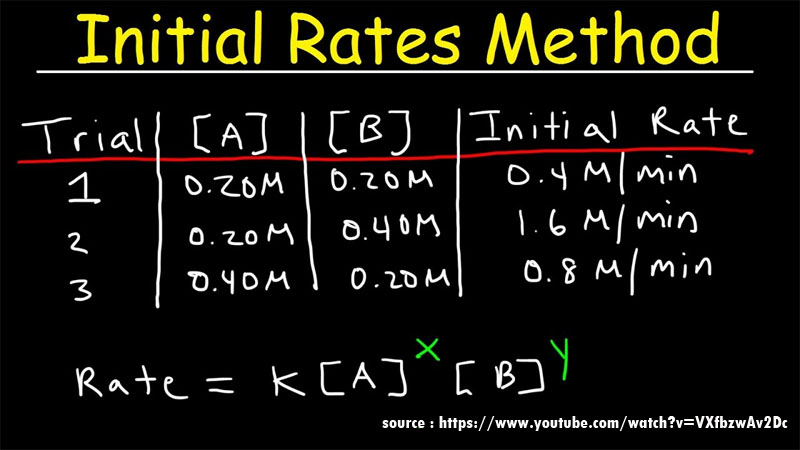# How To Know The Order Of The Reaction?Order of the reaction is the part of the chemical reaction which determines the relationship between the rate of a chemical reaction and the concentration of the species involved in the chemical reaction. Order of the reaction depends on the chemical concentration of the reactants involved in the chemical reaction.

The first-order reaction dependents entirely on the concentration of one of the reactants involved in the chemical reaction. Some characteristics of the reaction in order of reaction are listed below.

• The number of species of reactants involved along with the concentration of the reactants directly affects the rate of reaction.
• The value of the order of reaction can be in the form of a fraction or an integer. It can even have a value of zero.
• The order of a reaction is not dependent on the stoichiometric coefficients that are corresponding to each species in the balanced chemical reaction.
• It can be obtained by adding all the exponents of the concentration terms in the rate expression.
• The reaction order of a chemical reaction is defined with the help of reactant concentrations and not with product concentrations.

The rate of the reaction is determined by rate law or rate equation and is given by

r = k[A]x[B]y

Where,

[A] and [B] = concentration of the species involved in a chemical reaction

x and y = partial orders of reaction for A and B

r = rate of reaction

k= rate constant

Overall reaction = x+y

### Know The Energy Level Of Atoms And Molecules

As we know the atoms and molecules of the elements contains electrons. Electrons are the chief components responsible for determining the energy level of an atom. Electrons are assumed to 4 different energy levels that are used to describe the trajectory and the movement of an electron in an atom. This 4 numbers which represent the energy level of the electron is known as Quantum numbers. The quantum numbers of all the electrons in a given atom must comply with the Schrödinger wave equation for the hydrogen atom.

4 quantum numbers are described below:

• To (describes the energy level) principal quantum number = n
• azimuthal quantum number or Orbital angular momentum quantum number, denoted by l.
• Magnetic quantum number = m or ml.
• The electron spin quantum number = ms or s.

Quantum Number Values

According to the Pauli exclusion principle, no 2 electrons in an atom can have the same set of quantum numbers. Each quantum number is represented by a half-integer or integer value.

1. The principal quantum number is an integer that represents the number of the electron’s shell. The value shall not be 0 or negative and shall be 1 higher than 1
2. The angular momentum quantum number is an integer that is the value of the electron’s orbital (e.g., s=0, p=1). ℓ is greater than or equal to zero and less than or equal to n-1.
3. The magnetic quantum number represents the orientation of the orbital. They are usually integers ranging from -ℓ to ℓ. So, for the p orbital, where ℓ=1, m could have values of -1, 0, 1.
4. half-integer value that is either -1/2 or 1/2 is known as the spin quantum number.